# HSSlive: Plus One & Plus Two Notes & Solutions for Kerala State Board

## AP Board Class 7 Maths Chapter 5 Triangles InText Questions Textbook Solutions PDF: Download Andhra Pradesh Board STD 7th Maths Chapter 5 Triangles InText Questions Book AnswersAP Board Class 7 Maths Chapter 5 Triangles InText Questions Textbook Solutions PDF: Download Andhra Pradesh Board STD 7th Maths Chapter 5 Triangles InText Questions Book Answers

## Andhra Pradesh State Board Class 7th Maths Chapter 5 Triangles InText Questions Books Solutions

 Board AP Board Materials Textbook Solutions/Guide Format DOC/PDF Class 7th Subject Maths Chapters Maths Chapter 5 Triangles InText Questions Provider Hsslive

2. Click on the Andhra Pradesh Board Class 7th Maths Chapter 5 Triangles InText Questions Answers.
3. Look for your Andhra Pradesh Board STD 7th Maths Chapter 5 Triangles InText Questions Textbooks PDF.

## AP Board Class 7th Maths Chapter 5 Triangles InText Questions Textbooks Solutions with Answer PDF Download

Find below the list of all AP Board Class 7th Maths Chapter 5 Triangles InText Questions Textbook Solutions for PDF’s for you to download and prepare for the upcoming exams:

Question 1.
Classify the following triangles according to the length of their sides: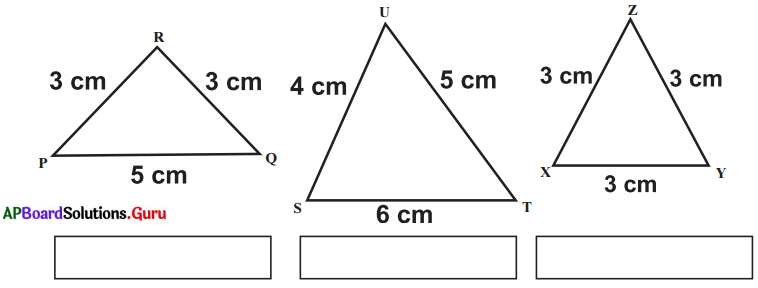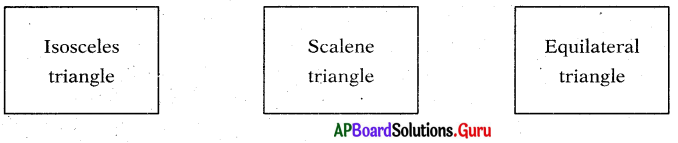[Page No. 93]

Question 2.
Classify the following triangles according to the measure of their angles.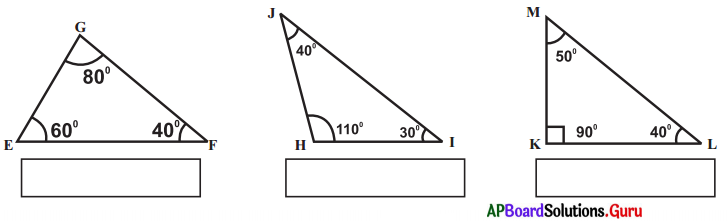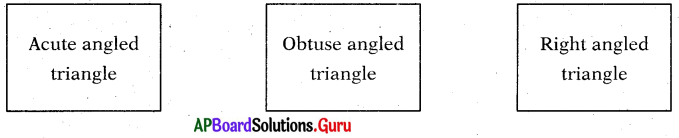Let’s Think [Page No. 97]

Question 1.
Can you find a triangle in which each angle is less than 60°?
Sum of angles in a triangle is 180°.
If each angle is less than 60°, then the sum of angles of a triangle is < 180°. So, triangle cannot form. So, we cannot find a triangle in which each angle is less than 60°.

Check Your Progress [Page No. 99]

Question 1.
Find the value of ‘x’ in the given figure.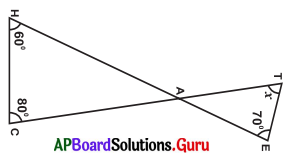In the given figure,
∠HAC = ∠TAE = a (∵ Vertically opposite angles)
In ∆AHC,
we know, ∠H + ∠C + ∠HAC 180°
⇒ 60° + 80° + a = 180°
⇒ 140° + a – 140° = 180°- 140° … a = 40°.
∴ ∠HAC = ∠TAE = a = 40°

In ∆ATE, we know,
∠T + ∠TAE + ∠E = 180°
⇒ x + a + 70° = 180°
⇒ x + 40° + 70° = 180°
⇒ x + 110°- 110° = 180°- 110°
∴ x = 70°

Let’s Do Activity [Page No. 101]

Question 1.
Measure the length of the sides of ∆ABC and fill the following table.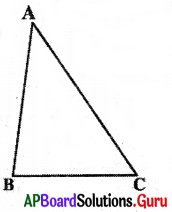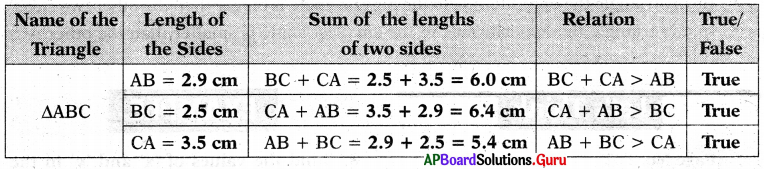From the above table, we can conclude that the sum of the lengths of any two sides of a triangle is greater than the length of the third side.

Question 2.
Take the same measurements of the previous activity and note the results as given below.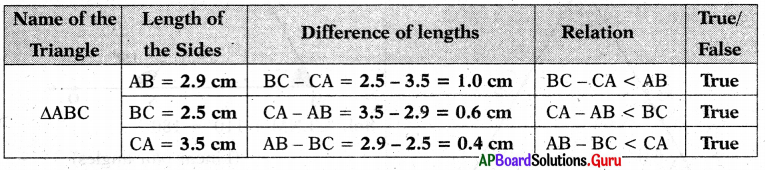From the above table we can conclude that the difference of the lengths of any two sides of a triangle is less than the length of the third side.

Question 3.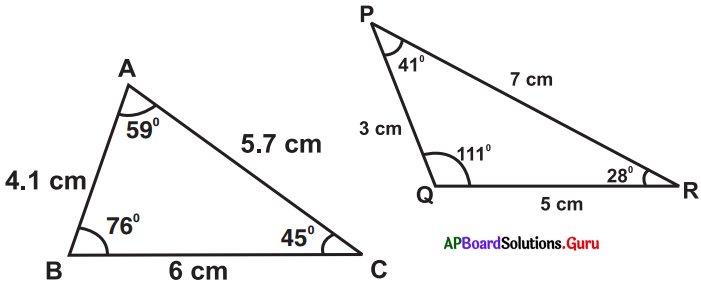Fill the following table as per measurements given in the above triangles: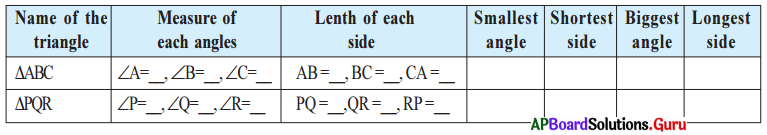What do you observe from the last four columns in above table ?

• In any triangle the opposite side to the biggest angle is bigger than the other two sides.
• In any triangle the opposite side to the smallest angle is smaller than the other two sides.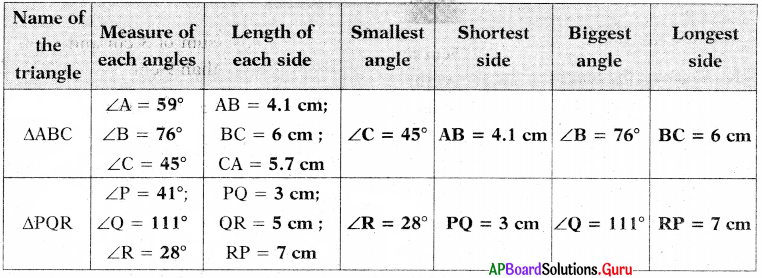Let’s Think [Page No. 102]

Question 1.
What are the measurements of angles of an equilateral triangle?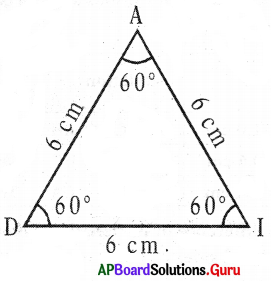AD = DI – AI = 6 cm (All angles are equal)
∠A = ∠D = ∠I = 60° (All angles are equal)
So, in an equilateral triangle,

All Sides are equal in length. Each angle is equal to 60°.

Puzzle Time [Page No. 104]

Question 1.
Find the interior angles of all triangles by using given dues.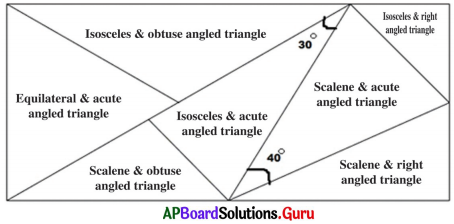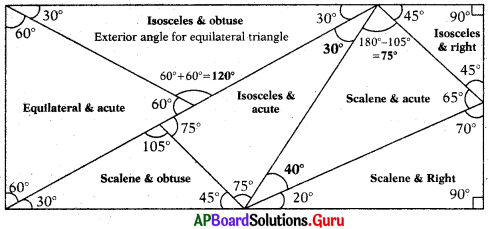Hints:
Each angle in an equilateral triangle is 60°.
Straight angle = 180°
In an isosceles triangle, two angles are equal.
In a scalene triangle all angles are unequal.
Right angle = 90°.
Obtuse angle > 90°.

Examples:

Question 1.
In a triangle, two angles are 43° and 57°. Find the third angle.
Given two angles of a triangle are 43° and 57°.
Sum of these two angles = 43° + 57° = 100°
In a triangle the sum of the interior angles is 180°.
∴ Third angle = 180° – 100° = 80°

Question 2.
Find the value of ‘x’ in the following triangles :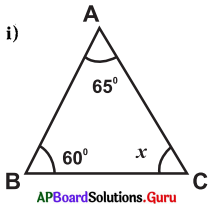From the figure
∠A + ∠B + ∠C = 180° (v Sum of three angles in triangle is 180°)
⇒ 65° + 60° + x = 180°
⇒ 125° + x = 180°
∴ x =180°- 125° = 55°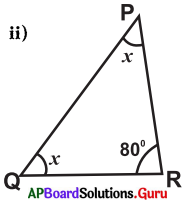From the figure
∠P + ∠Q + ∠R = 180°
x + x + 80° = 180°
⇒ 2x + 80° = 180°
⇒ 2x = 180°-80°
⇒ 2x = 100°
⇒ x = 1002 = 50°
∴ x = 50°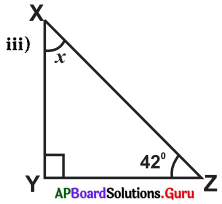From the figure
∠X + ∠Y + ∠Z – 180°
⇒ x + 90° + 42° = 180°
⇒ x + 132° = 180°
⇒ x = 180°- 132°
∴ x = 48°

Question 3.
Find the values of x and y in the given triangle.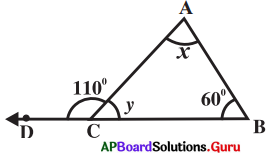In the ∆ABC
∠ACD + ∠ACB = 180° (linear pair of angles)
110° + y = 180°
⇒ y = 180°- 110°
∴ y = 70° ……………….(i)

∠BAC + ∠ACB + ∠CBA = 180°
⇒ x + y + 60° = 180°
⇒ x + 70° + 60° = 180° (From (i))
⇒ x + 130° = 180°
∴ x = 50°

Question 4.
In a right angled triangle one acute angle is 44°, then find the other acute angle.
We know that, sum of the two acute angles in a right angled triangle is 90°.
Given that, in the right angled tri-angle one of the acute angles = 44°
Other acute angle in right angled triangle = 90° – 44° = 46°

Question 5.
The angles of a triangle are (x + 10)°, (x – 20)° and (x + 40)°. Find the value of x and the measure of the angles.
Given that the angles of the triangle are
(x + 10)°, (x – 20)° and (x + 40)°
(x + 10)° + (x – 20)° + (x + 40)° = 180°
⇒ x + 10° + x – 20° + x + 40° = 180°
⇒ 3x + 30° = 180°
⇒ 3x – 180° – 30°
⇒ 3x = 150°
⇒ x = 1503 = 50°

The angles are,
x + 10° = 50° + 10° = 60°
x – 20° = 50° – 20° = 30°
x + 40° = 50° + 40° = 90°
∴ Measure of the angles are 60°, 30° and 90°.

Question 6.
In the ∆ABC, exterior angle at ∠C = 105° and ∠A=65°. Find the other interior opposite angle.
Given that, exterior angle at ∠C = 105°.
One of the interior opposite angle ∠A = 65°.

The other interior opposite angle is ∠B .
∠A + ∠B =105°(∵ Exterior angle property of a triangle)
65° + ∠B = 105°
⇒ ∠B = 105° – 65° = 40°

Question 7.
Find the exterior angle of the given triangle.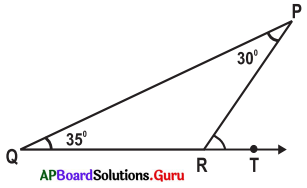From the figure, ∠P = 30°, ∠Q = 35°
Exterior angle at R = ∠P + ∠Q (∵ Exterior angle property of a triangle)
= 30° + 35° = 65°

Question 8.
Find the values of ‘x’ and ‘y’ in the following figure.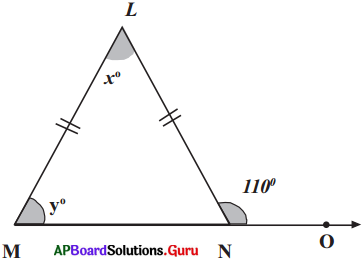∠LMN + 110° = 180° (Linear pair angles)
∠LNM = 180° -110° = 70°
∠LMN = ∠LNM (Angles opposite to equal sides)
y = 70°
⇒ x + y – 110° (Exterior angle property)
⇒ x + 70° = 110°
∴ x = 110°-70° – 40°

## Andhra Pradesh Board Class 7th Maths Chapter 5 Triangles InText Questions Textbooks for Exam Preparations

Andhra Pradesh Board Class 7th Maths Chapter 5 Triangles InText Questions Textbook Solutions can be of great help in your Andhra Pradesh Board Class 7th Maths Chapter 5 Triangles InText Questions exam preparation. The AP Board STD 7th Maths Chapter 5 Triangles InText Questions Textbooks study material, used with the English medium textbooks, can help you complete the entire Class 7th Maths Chapter 5 Triangles InText Questions Books State Board syllabus with maximum efficiency.

## FAQs Regarding Andhra Pradesh Board Class 7th Maths Chapter 5 Triangles InText Questions Textbook Solutions

#### Can we get a Andhra Pradesh State Board Book PDF for all Classes?

Yes you can get Andhra Pradesh Board Text Book PDF for all classes using the links provided in the above article.

## Important Terms

Andhra Pradesh Board Class 7th Maths Chapter 5 Triangles InText Questions, AP Board Class 7th Maths Chapter 5 Triangles InText Questions Textbooks, Andhra Pradesh State Board Class 7th Maths Chapter 5 Triangles InText Questions, Andhra Pradesh State Board Class 7th Maths Chapter 5 Triangles InText Questions Textbook solutions, AP Board Class 7th Maths Chapter 5 Triangles InText Questions Textbooks Solutions, Andhra Pradesh Board STD 7th Maths Chapter 5 Triangles InText Questions, AP Board STD 7th Maths Chapter 5 Triangles InText Questions Textbooks, Andhra Pradesh State Board STD 7th Maths Chapter 5 Triangles InText Questions, Andhra Pradesh State Board STD 7th Maths Chapter 5 Triangles InText Questions Textbook solutions, AP Board STD 7th Maths Chapter 5 Triangles InText Questions Textbooks Solutions,
Share: